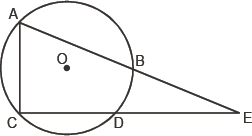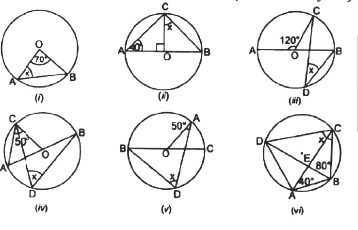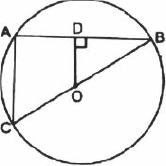1800-212-7858 (Toll Free)
9:00am - 8:00pm IST all days
8104911739

or

Thanks, You will receive a call shortly.
Customer Support

You are very important to us

For any content/service related issues please contact on this toll free number

022-62211530

Mon to Sat - 11 AM to 8 PM

Ask Properties Of Equal Chords question free ×

Queries asked on Sunday and after 7 pm from Monday to Saturday will be answered after 12 pm the next working day.

• CBSE×
• Class 9×
• Mathematics×
• Circles×
• Properties Of Equal Chords×

# Properties Of Equal Chords Free Doubts and Solutions

## BE and CD are two equal chords of circle with centre O. These chords when produced meet at E. Prove that BE = ED .

Asked by Vikas 11th January 2018, 9:10 AM

×

## 1.In the figure, OD is perpendicular to chord AB of a circle whose centre is O. If BC is diameter; prove that CA = 2OD. 2.   l is a line intersecting two concentric circles having common centre O, at A, B, C and D. Prove that AB = CD. 3.   AB and CD are equal chords of a circle whose centre is O. When produced, these chords meet at E. Prove that EB = ED.4.   If O be the centre of the circle, find the value of ï¿½xï¿½ in each of the following figures.5.   Prove that equal chords of a circle subtend equal angles at the centre. 6.   The line drawn through the centre of a circle to bisect a chord is perpendicular to the chord. Prove it. 7.   Prove that equal chords of a circle (or congruent circles) are equidistant from the centre (or centres). 8.   In the figure, OD is perpendicular to the chord AB of a circle with centre O. If BC is a diameter, show that AC || OD and AC = 20D.Hint: ∴ OD ⊥ AB               therefore; D is the mid-point of AB. 9.   If two intersecting chords of a circle make equal angles with the diameter passing through their point of intersection, prove that the chords are equal. 10.   Show that the angles in the same segment of a circle are equal.

Asked by aahnik.mohanty 30th December 2017, 10:21 AM

×

## O is the centre of the circle, find the value of x.

Asked by Topperlearning User 5th October 2017, 8:25 AM

×

## In the figure, O is the centre of a circle. Prove that x + y = z.

Asked by Topperlearning User 5th October 2017, 8:24 AM

×

## O is the centre of the circle and PQ is a diameter. IfROS is equal to 40o findRTQ.Asked by Topperlearning User 5th October 2017, 8:22 AM

×

## P is the centre of the circle. Prove thatXPZ is equal to twice the sum ofXZY andYXZ.Asked by Topperlearning User 5th October 2017, 8:17 AM

×

## Prove that the angle in the minor segment is an obtuse angle.

Asked by Topperlearning User 5th October 2017, 8:15 AM

×

## In the given figure, equal chords AB and CD of a circle C(O, r) cut at right angles at E. If M and N are the mid points of AB and CD respectively, prove that OMEN is a square.

Asked by Topperlearning User 18th August 2017, 8:51 AM

×

## AB and AC are two equal chords of a circle whose centre is O. If OD is perpendicular to AB and OE is perpendicular to AC, prove thatADE is an isosceles triangle.Asked by Topperlearning User 18th August 2017, 8:49 AM

×

## PQ and RQ are the two chords of a circle equidistant from the centre O. Prove that the diameter passing through Q bisectsPQR andPSR.Asked by Topperlearning User 18th August 2017, 8:40 AM

×

## TWO EQUAL CHORDS AB AND CD OF A CIRCLE WHEN PRODUCED INTERSECT AT A POINT P.PROVE THAT PB=PD.

Asked by vrishti TOKAS 22nd February 2016, 8:09 PM

×

## Q1. Prove that the quadrilateral formed by the internal angle bisectors of any quadrilateral is cyclic ?   Q2. AB is a diametre of the circle ,CD is a chord equal to radius of the circles ,AC and BD when extended intersect at point E. Prove that angle AEB=60 ?   Q3. A chord of a circle is equal to radius of the circle. Find the angle subtended by the chord at a point on the minor arc and also at a point on a major arc ?   Q4. If the non-parallel sides of a trapezium are equal ,prove it is cyclic ?

Asked by shub3893 3rd December 2015, 8:56 PM

×

## Given is AB the diameter of circle and CD is a chord equal to the radius of the circle. AC & BD when extended intersect at a point E.Then Prove that Angle AEB = 60.

Asked by singhaivk05 25th February 2015, 5:33 PM

×

## Please Explain NCERT Textbook, exercise 10.4 , Q5 and Q6.

Asked by Radha Pandey 5th January 2015, 1:29 PM

×

## Two equal chords AB and CD of a circle when produced, intersect at point P. Prove that PB = PD.

Asked by Topperlearning User 4th June 2014, 1:23 PM

×

## In the figure, O is the centre of the circle, OM BC, OL AB, ON AC and OM = ON = OL. Is ABC equilateral? Given reasons.

Asked by Topperlearning User 4th June 2014, 1:23 PM

×

## In the figure A, B and C are three points on a circle such that the angle subtended by the chords AB and AC at the centre are 120o and 80o respectively. Determine angle BAC.

Asked by Topperlearning User 4th June 2014, 1:23 PM

×

## If two intersecting chords PQ and RS of a circle make equal angles with the diameter passing through their point of intersection, then prove that the chords are equal.

Asked by Topperlearning User 4th June 2014, 1:23 PM

## Answer this question

×

### CBSE - IX - Mathematics - Circles

Asked by Topperlearning User 11th February 2014, 9:10 AM

## Answer this question

×

### CBSE - IX - Mathematics - Circles

Asked by Topperlearning User 7th February 2014, 4:10 PM

×

## Two equal chords of a circle intersect within the circle; prove that the line joining the point of intersection to the centre makes equal angles with the chords.

Asked by Topperlearning User 7th February 2014, 3:55 PM

×

## Two congruent circles interest each other at points A and B. Through point A, line segment PAQ is drawn so that P and Q lie on the two circles. Prove that BP = BQ.

Asked by Topperlearning User 7th February 2014, 12:39 PM

×

## If two intersecting chord of a circle make equal angles with the diameter passing through their point of intersection, prove that the chords are equal.

Asked by Topperlearning User 7th February 2014, 12:36 PM

×

## In the figure, AB and CD are two equal chords of a circle with centre O. OP and OQ are perpendiculars on chords AB and CD respectively. If POQ = 150o, find APQ.

Asked by Topperlearning User 7th February 2014, 12:35 PM

×

## The two intersecting chords of a circle make equal angles with the diameter passing through their point of intersection. Prove that the chords are equal.

Asked by Topperlearning User 7th February 2014, 12:33 PM